# Array Representation by Compiler

## Array Representation by Compiler in C and C++

In this article, I am going to discuss Array Representation by Compiler i.e. how a Compiler Handles or Manages Arrays in C and C++. Please read our previous article, where we discussed 2-Dimensional Array in C and C++. Here, we will discuss how a single-dimensional array is represented by a compiler as well as how a two-dimensional and n-dimensional array is represented using both Row Major order and Column Major order.

##### How a scalar Variable is represented by the compiler?

Before understanding how the compiler handles an array, let us first understand how the compiler handles a simple variable that is a scaler variable. In our programs, we use variables as names for representing some data, for example, int x = 10;

But when the compiler converts it into machine code, machine code will note have variable names. During execution for this variable (x), let us say two bytes of memory are allocated and the address is 1000 and 1001. Here, during execution, the variable X represents the memory address (1000/1002) and the value 10 has to be stored at that memory location i.e. at that address 1000/1001. For better understanding, please have a look at the below image.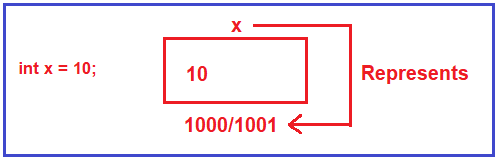So, basically, the machine code should refer to the location with the address, not by the variable name. Then compiler has to convert the name into an address. When the address will be known? When the memories are located and when the memories are located? During the execution of the program. It means the memory for the variable is allocated at execution and then only the address can be known. Then how the compiler will write down the address at compiler time? so, that’s what we will learn about that issue in the array.

Please have a look at the below image. Here we have an array (i.e. A) that is initialized with some values (i.e. 1, 3, 5, 7, and 9). During execution time, the memory will be allocated for the array and the values will be stored at their corresponding locations.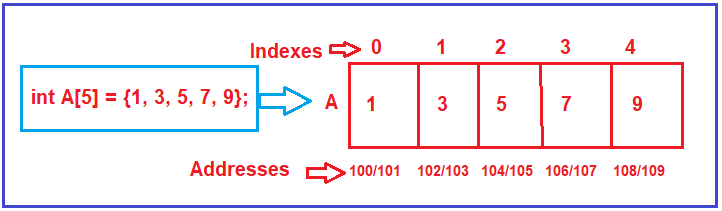Now, in our program, if we want to store a value 10 in the fourth location i.e. at the index position 3, then we need to write the below code.

A = 10;

The above is a statement in our program which should be converted into machine code which is referred to by address 106. So, the compiler has to refer to that location that is 106.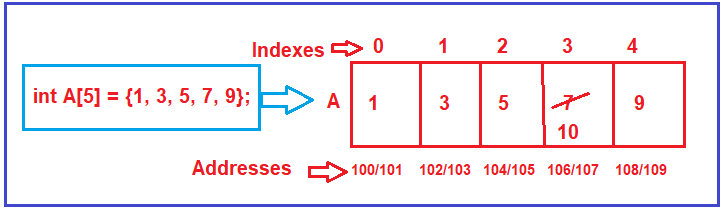##### How the code (A =10;) of our program will get converted into machine code?

Actually, the compiler needs the address of the location A i.e. address(A). At compile time the address cannot be known. So, the compiler will write down a formula for obtaining the address. Let us see how that formula looks like.

The name of the array is A and 100 is the first address of the array. Let’s can call it L0 (i.e. Location of 0). The first address of the array is called the base address of the array. Any location of the array can be accessed with the help of the base address. So, L0 (i.e. Location of 0) is the base address that is 100. Which location (i.e. the index position) you want to access? 3rd index position. How many bytes does integer take? Let’s assume 2 bytes. Then the formula for accessing the 3rd index (fourth location) is given below.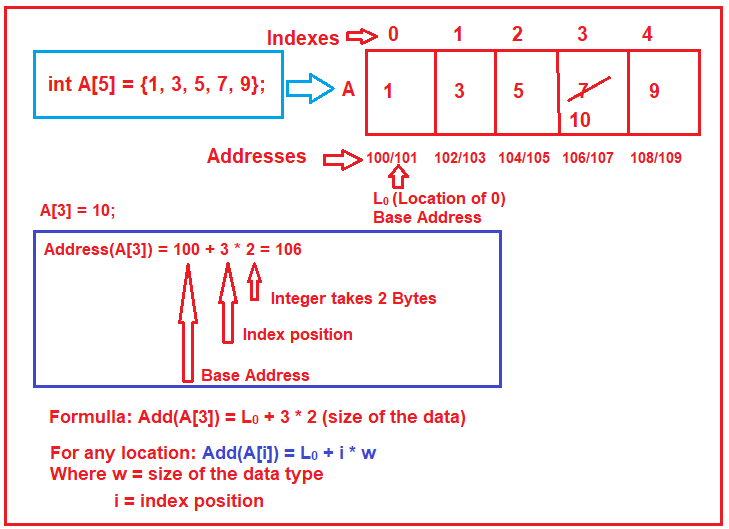This is the formula used by the compiler for converting any index to an address. Now, for obtaining the actual address during runtime our program must know the base address for an array. The base address of an array will be updated once the program starts running and once the memory for the array is allocated. So, the base address of the array is known during runtime and it is updated at runtime and this concept is called data binding.

If the Index position of an array starts from 1 instead of 0, then what should be the formula? For this please have a look at the below code.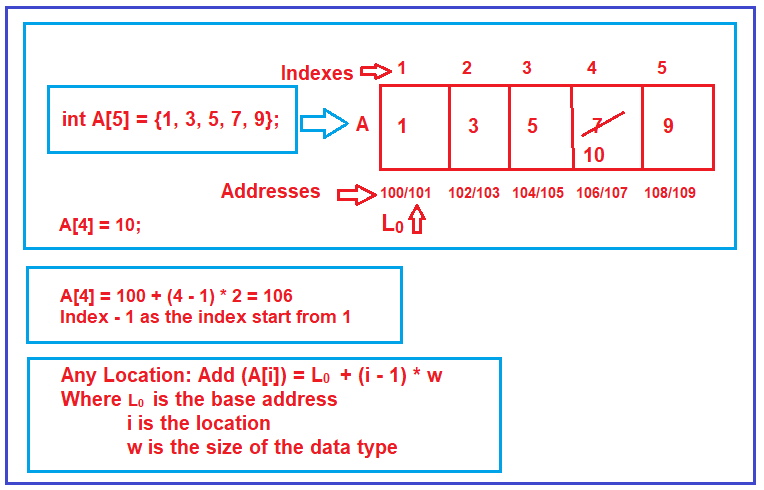The above one is the formula in the indexes starting from 1 onwards. This is not there in C and C++ but this is used to be there in older languages. In older programming languages they used to allow the developer to declare an array starting from any index. But in C and C++ it is strictly from 0 only.

##### Why array index is strictly to start with 0 in C and C++?

In order to understand this, we need to compare the formula for calculating the index when starting with 0 and 1. We already discussed both the formula and which is shown in the below image. When the array index starts with 0, the number of operations will be 2 (i.e. one addition and one multiplication) to calculate the address. On the other hand, when the array index starts with 1, the number of arithmetic operations will be 3 (i.e. addition, minus, and multiplication) to calculate the address of a location.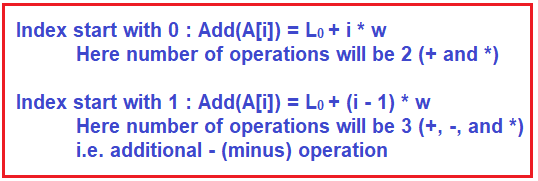So, if you have a big array if the array index starts with 1, then for each address calculation, it will take some time as compared to when the array index starts with 0. This is the reason why to eliminate that extra time (i.e. that minus operation), it is strictly in C and C++ to starts the array index with 0.

##### How a two-dimensional array is managed or handled by a Compiler in C/C++?

We have discussed how a single-dimensional array is managed by Compiler. Now let us proceed and understand how a two-dimensional array is managed or handled by the compiler in C and C++. Let us understand this concept with examples.

##### Understanding how a two-dimensional array is managed by Compiler

The following is syntax two declare a two-dimensional array in C/C++. Here, m represents the number of rows and n represents the number of columns

int A[m][m]; For example, if you want to declare a two-dimensional with 3 rows and 4 columns, then the syntax must be something like below.

int A;

Then the following image shows how the above two-dimensional (3 rows and four columns) array is represented on paper. So, any element of a two-dimensional array can be accessed with the help of its row number and column number.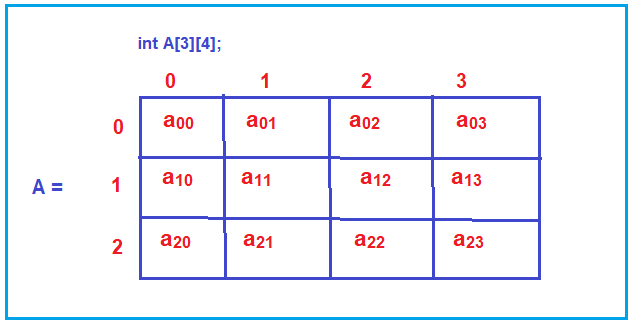But the reality is, during the execution, actual memory allocation is linear i.e. like a 1-Dimensional array. It will not be in terms of rows and columns but it will be like a single-dimensional array as shown in the below image. So, a total of three rows and four columns i.e. (3 * 12 = 12) locations will be there of type whatever the array type you mentioned. In our example, the array type is an integer and we assume integer takes two bytes and also assuming that the starting address or base address is 100.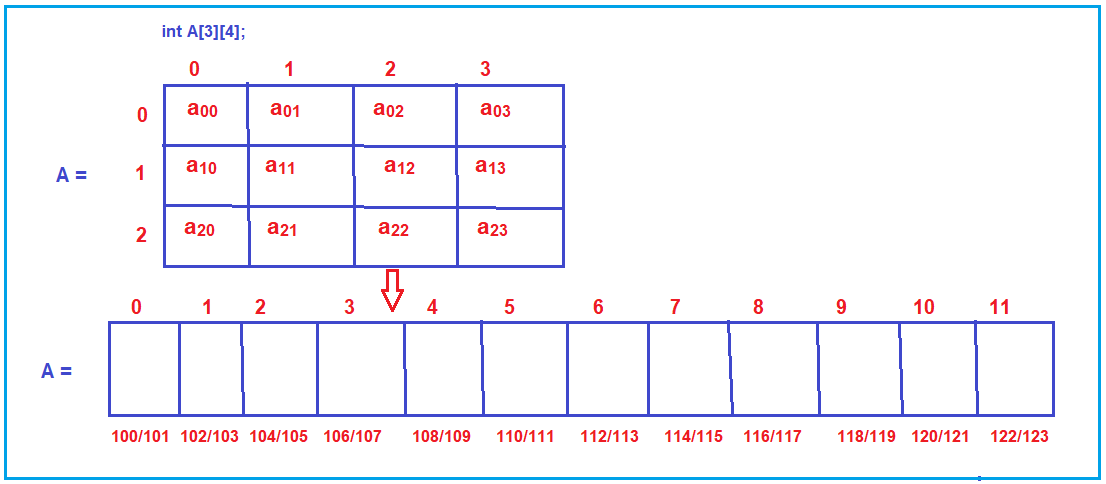Now the question is how the elements of a two-dimensional array stored in a single dimensional array or how the elements of a two-dimensional array mapped to a single dimensional array. There are two methods for mapping or representation a two-dimensional array into the one-dimensional array in C and C++. They are as follows:

1. Row Major Mapping
2. Column Major Mapping
##### How Row Major Mapping is done in C and C++?

Let us understand how row-major mapping is done in C and C++ to map a two-dimensional array into a single-dimensional array. In this case, the elements of the two-dimensional array are stored in the single-dimensional array row by row. For better understanding, please have a look at the below image. So, in Row major mapping the elements are stored row by row.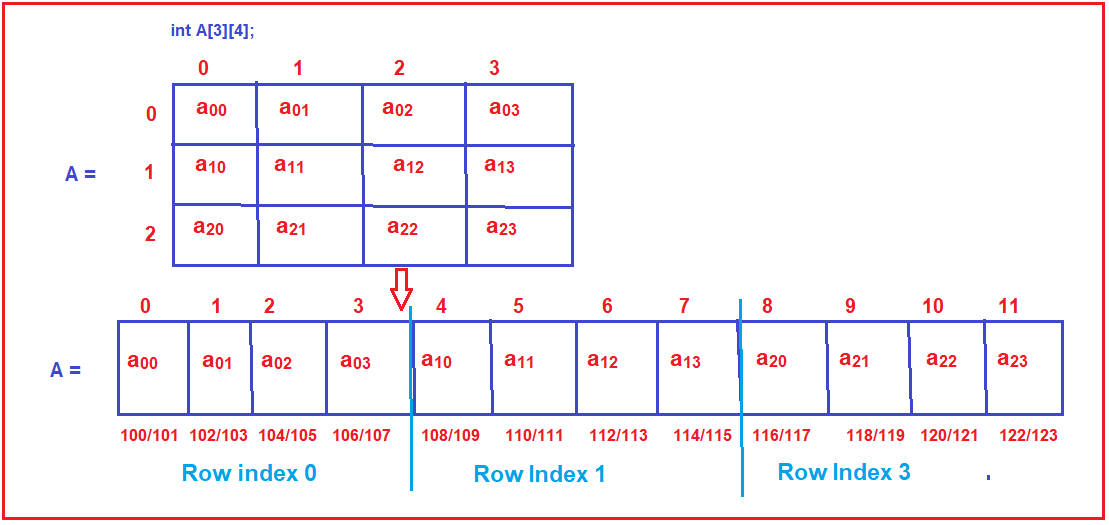As you can see in the above image, it will store the elements row by row. That means it will take the first four elements (the row with index 0) of the two-dimensional array and stored them into the single-dimensional array. Then take the next four elements (i.e. the second-row element) and stored them into the single-dimensional array. Finally, takes the last four elements (i.e. row index 2) and store them in the single-dimensional array, that’s what you see in the above image. This is Row Major order Mapping.

##### What is the formula used by the compiler to calculate the address of a location when the elements are stored in Row Major Order?

Now let us see the formula used by the compiler to calculate the address of a location when the elements are stored in Row Major Order. Let say we want to access the elements which are present in A i.e. in the two-dimensional array it is the 2nd row and third column. If you notice in the single-dimensional array, it is in the index position 6 with the address 112/113.

Suppose we want to store a value 10 in A, then that A has to be converted into an address by the compiler. As we already discussed addresses are not known at compiler time, what the compiler does is, writes down one formula for obtaining the address. So, let us observe what should be the formula. For this please have a look at the following image.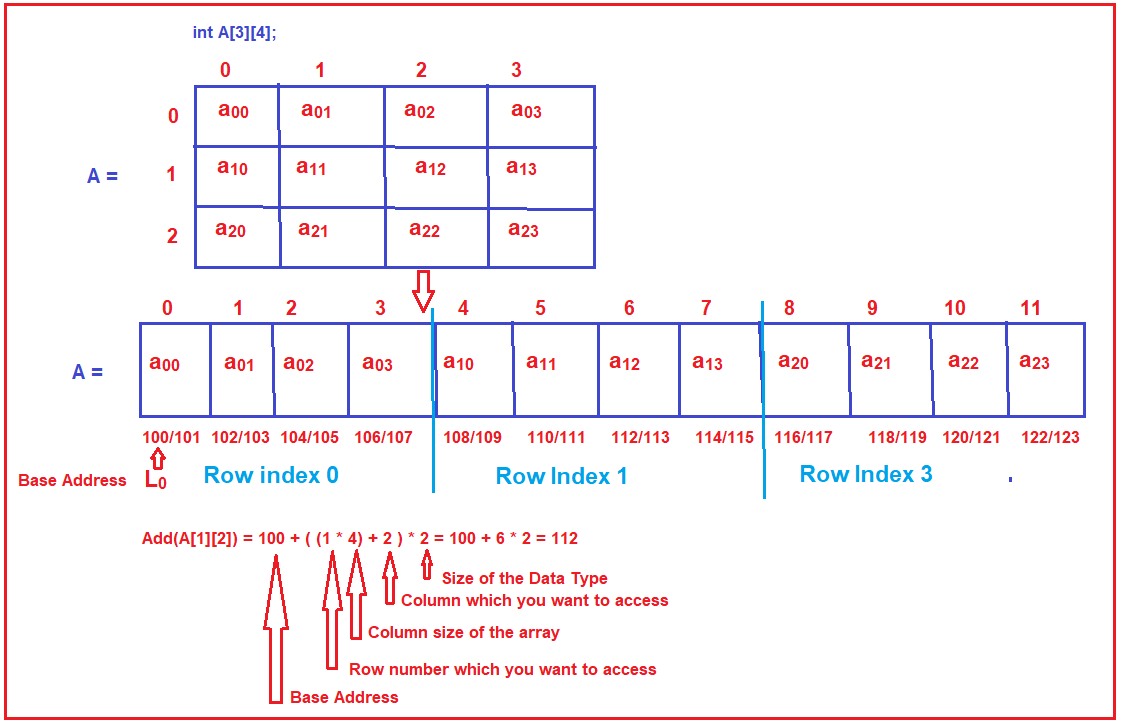So, if you want to access A, then the following is the formula in Row Major order

A = 200 + ((1 * 4 ) + 2) * 2 = 100 + 6 * 2 = 112

From this we can create a formula for calculating the address of an array location in Row Major order as follows: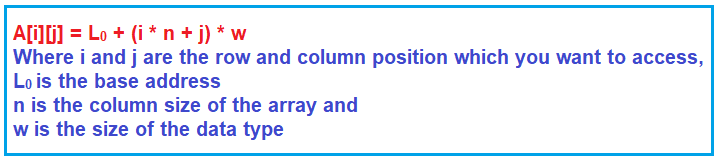Note: The two-dimensional arrays are accessed using two indexes but actually they are kept in a single-dimensional array that needs just one index.

##### If the Language allows starting the index from 1 onwards then what should be the formula?

Following is the formula, when the array index starts with 1.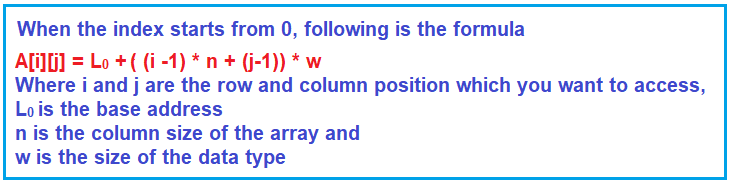If you see, if the index starts from 1, in Row Major representation, the number of arithmetic operations will be 6 compared to the number of operations 4 when the index starts with 0. This is the reason why the array index starts with 0 in C and C++ Language when it represents the two-dimensional array using Row Major order.

##### How Column Major Mapping is done in C and C++?

Let us understand how Column major order mapping is done in C and C++. In this case, the elements of the two-dimensional array are stored in the single-dimensional array column by column. For better understanding, please have a look at the below image. In Column major order mapping the elements are stored column by column.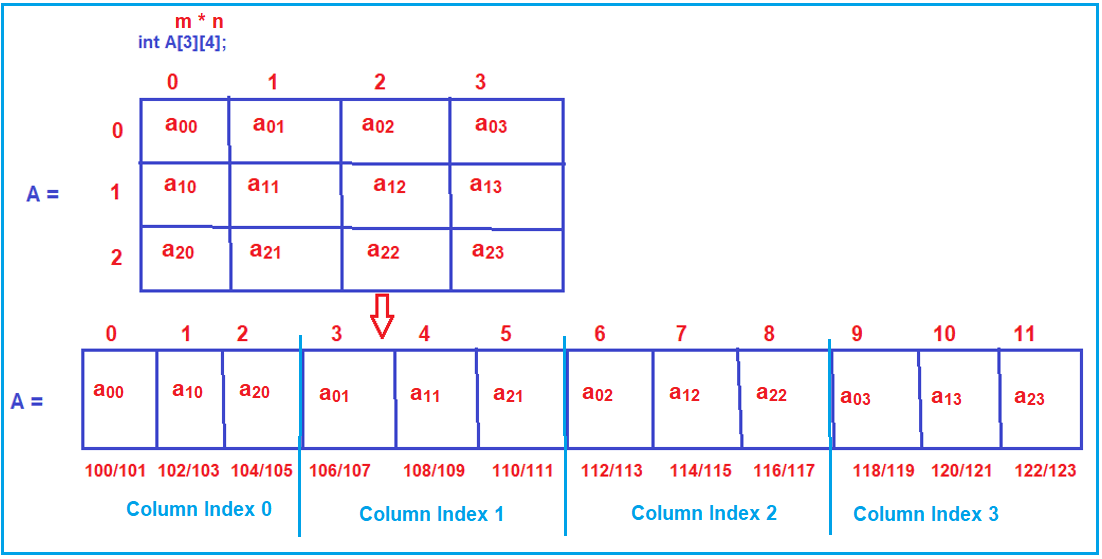As you can see in the above image, it will store the elements column by column. That means it will take the first three elements (column with index 0) of the two-dimensional array and stored them into the single-dimensional array. Then take the next three elements (i.e. the second column elements) and stored them into the single-dimensional array. Then, takes the next three elements (i.e. column index 2) and store them in the single-dimensional array, and finally, takes the fourth column elements and store them into the single-dimensional array, that’s what you see in the above image. This is Column Major order Mapping.

##### What is the formula used by the compiler to calculate the address of a location when the elements are stored in Column Major Order?

Now let us see the formula used by the compiler to calculate the address of a location when the elements are stored in Column Major Order. Let say we want to access the elements which are present in A i.e. in the two-dimensional array it is the 2nd row and third column. If you notice in the single-dimensional array, it is in the index position 7 with the address 114/115.

Suppose we want to store a value 10 in A, then that A has to be converted into an address by the compiler. As we already discussed addresses are not known at compiler time, what the compiler does is, writes down one formula for obtaining that address. So, let us see what should be the formula when the elements are stored using column-major order. For this please have a look at the following image.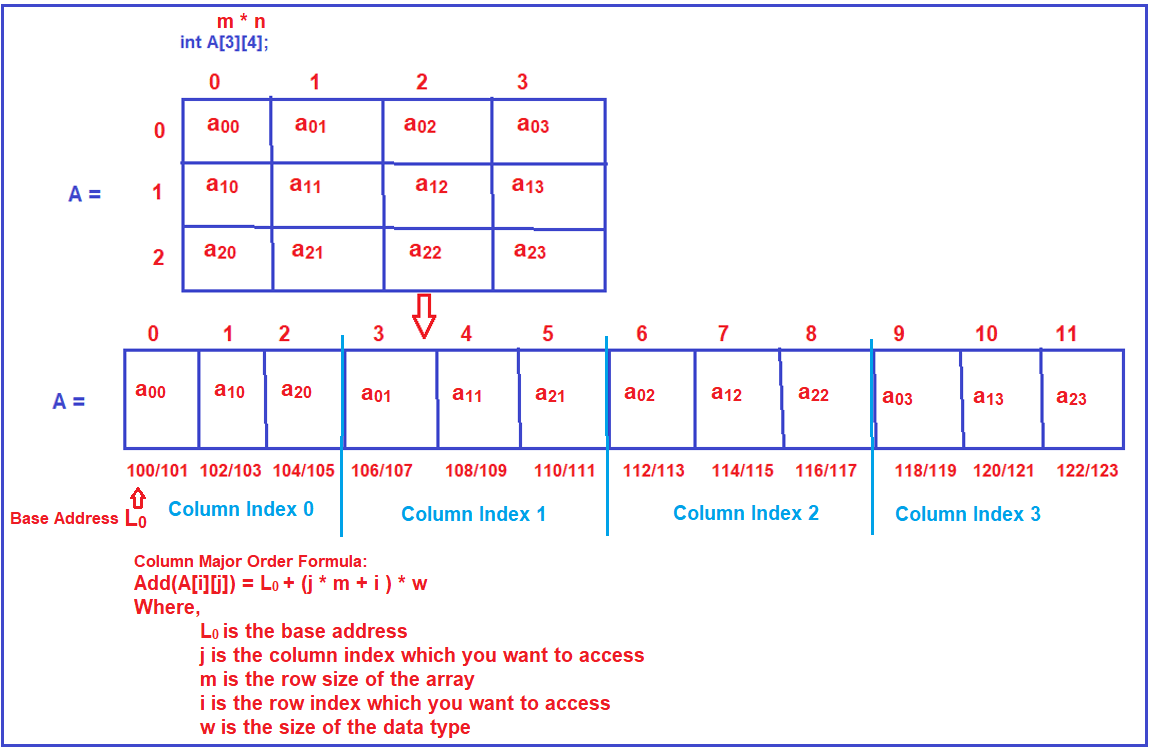Let us see some examples.
A = 100 + (2 * 3 + 1) * 2 = 100 + (6 + 1) * 2 = 100 + 7 * 2 = 114 (Correct address, you can see in the image)
A = 100 + (3 * 3 + 2) * 2 = 100 + (9 + 2) * 2 = 100 + 11 * 2 = 122 114 (Correct address, you can see in the image)

Note: If the indexes are starting from 1 onwards, then simply you need to use -1 in I and J.

##### Important Point:

Both the formulas Row Major Order and Column Major order are having the same number of operations and hence in terms of time both are equally efficient. So, we can’t say one formula is better than the other. It’s the compiler who will choose what formula to use. If you are designing your own compiler, then you can choose whatever formula you want to use. But C and C++ follow Row Major Formula for representing a two-dimensional array.

##### The formula for n-Dimensional Array:

Let us prepare a row-major formula and column-major formula for a four-dimension array. The following is an example of a four-dimensional array. Here, the name of the array is A and the data type is anything (it can be an integer, string, float, etc.) and dimensions are d1, d2, d3, and d4.

Type A[d1][d2][d3][d4]

Please have a look at the following, for the address of any location whose indices are i1, i2, i3, and i4 then what should be the formula.

##### Row Major Formula for a 4-Dimensional Array:

Let us first prepare the formula for row-major. Following is the formula for a 4-dimensional array in row-major order.
Add (A[i1][i2][i3][i4]) = L0 + [i1*d2*d3*d4 + i2*d3*d4 + i3*d4 + i4] * w
Where,

For i1 = multiply i1 with the dimension by leaving the first dimension i.e. i1*d2*d3*d4
For i2 = multiply i2 with the dimension by leaving the first & second dimension i.e. i2*d3*d4
For i3 = multiply i3 with the dimension by leaving the first, second & third dimension i.e. i3*d4
For i4 = multiply i4 with the dimension by leaving the first, second, third & fourth dimension i.e. i3 (no dimensional to multiply)

w = size of the datatype

This is the approach that we have to use to calculate the row-major order formula for 1 four-dimensional array. If you observe the Row Major formula, we are going from left to right as shown in the below image.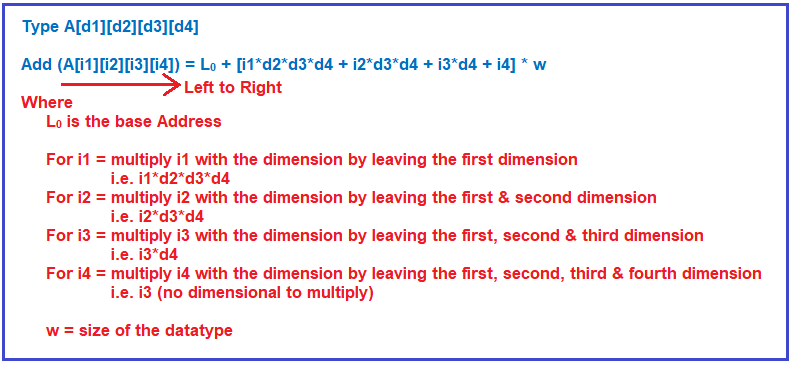##### Column Major Formula for 4D Array:

Let us see how to prepare the formula for column-major order. Following is the formula for a 4-dimensional array in column-major order. In column-major order, we need to go from right to left,
Add (A[i1][i2][i3][i4]) = L0 + [i4*d1*d2*d3 + i3*d1*d2 + i2*d1 + i1] * w
Where,
For i4 = take all the dimensions which are present before d4 and multiple with i4 i.e. i4*d1*d2*d3
For i3 = take all the dimensions which are present before d3 and multiple with i3 i.e. i3*d1*d2
For i2 = take all the dimensions which are present before d2 and multiple with i2 i.e. i2*d1
For i1 = take all the dimensions which are present before d1 and multiple with i1 i.e. i1 (no dimension present)
w = size of the datatype

This is the approach that we have to use to calculate the column-major order formula for 1 four-dimensional array. If you observe in the Column Major formula, we are going from right to the left, as shown in the below image.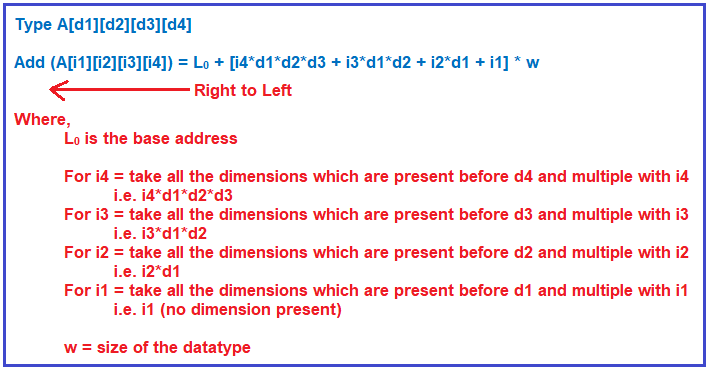Using a 4Dimensional array, I have shown you the formula to write the row-major and column-major formula. By using the same approach, now we can write the formula for any dimensions, even a 2Dimensional array was following the same approach. Now, based on these formulas we can prepare a general form of this one for n dimensions. So, let us prepare for a general formula for n dimension. Let us prepare a general formula for n dimensions for row-major mapping.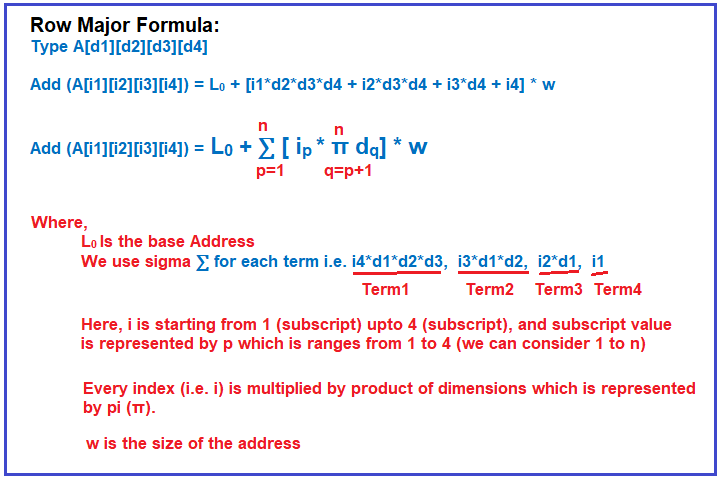Now, it is a task for you to prepare the formula for column-major order and put the formula explanation in the comment section of this article.

Now, let us try to find out the time complexity of the above formula. We are going to analyze the row-major formula. Please have a look at the below image.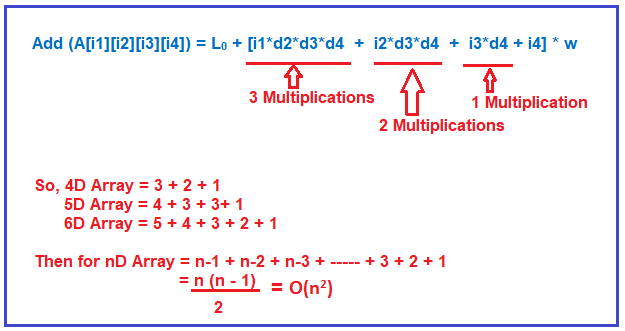So, the time taken for the above formula is n2 multiplication operations. This is too much time-consuming. The row-major formula used by the compiler to take the order of n2 time. Is there any way to reduce the number of multiplications? Let us see what we can do for reducing the number of multiplications.

##### Reducing the Number of Multiplications:

Let us see how we can reduce the number of multiplications. Here, I am only taking the formula which is inside the bracket and will write the formula from right to left as shown in the below image.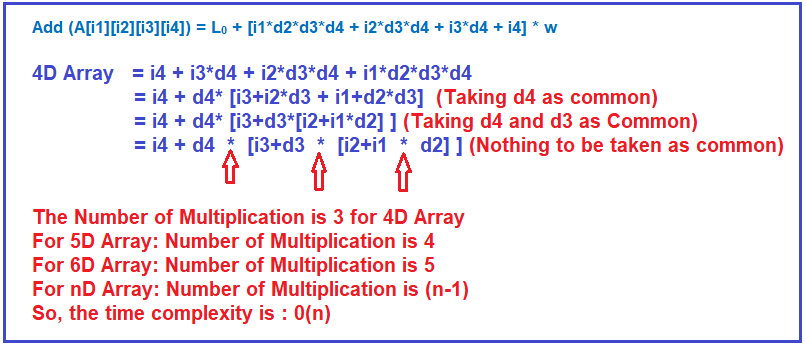As you can with the above-optimized formula the number of multiplications will be n-1 and hence the time complexity will be the order of n i.e. O(n). So, we have seen by taking common how we reduced the number of multiplications from n2 to n-1. The rule that we applied here to reduce the number of multiplications is called Harner’s rule.

The task for You: Now it is a task for you to calculate the formula for 3D Array using both row-major and column-major order representation by Compiler.

In the next article, I am going to discuss Array Quiz. Here, in this article, I try to explain how a two-dimensional array is represented by the compiler using both Row Major Order and Column Major order. I hope you enjoy this two-dimensional array representation article. Please give your feedback, suggestions, and comments about this article.